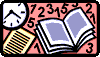Simple operations 3Tables of addition - - - - - - - - - - Tables of subtraction - - - - - - - - - -

Instructions: Press one of the buttons on the right column The correct number is put in red .

 2 + 8 = 4 + 7 = 6 + 5 = 8 + 4 = 10 + 8 = 8 - 3 = 15 - 5 = 14 - 7 13 - 9 18 - 11 = 3 + 1 = 5 + 5 = 7 + 6 = 9 + 4 = 11 + 6 = 4 - 2 = 13 - 4 = 11 - 6 = 10 - 8 = 17 - 10 = 5 - 3 = 4 + 5 = 6 + 7 = 8 + 8 = 10 + 4 = 2 + 1 = 9 - 5 = 12 - 7 = 17 - 9 = 16 - 11 = 12 - 2 = 3 + 6 = 5 + 2 = 7 + 8 = 9 + 8 = 11 + 8 = 11 - 4 = 8 - 6 = 18 - 8 = 15 - 10 =